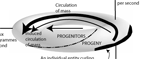﻿ Overview | Engenetics: The science of species

THE FIVE PROPOSITIONS
This web site uses the four fundamental theorems of the vector calculus,
in conjunction with an experiment conducted with Brassica rapa,
to prove the following five propositions, and to create a relativistic quantum biology:

• PROPOSITION I

That biology is “the study of those systems that can replace their internal energy”; that it is described by the general equation dU = Mdt = δQ - dH; M > 0 (see explanation of variables and terms); and that it is governed by the four laws of biology summarized upon the left.
• PROPOSITION II

That ecology is “the study of the processes systems use to replace their internal energy”; that it is described by the equation pdt + mdt = dh + du = δq; m > 0 (see explanation of variables and terms); and that it is governed by the four maxims of ecology summarized upon the right.
• PROPOSITION V

(A)
That the Gibbs-Duhem equation

m̅μ = dS = dU + dH - Σi μi(dvi - dmi)
governs all biological energy;

(B) That the Euler equation

μ = dS = (∂S/∂U)V,Ni dU + (∂S/∂V)U,Ni dV + Σi (∂S/∂ui)U,V,{Nj≠i} dui + Σi (∂S/∂vi)U,V,{Nj≠i} dvi
governs all biological activity;

(C) That it is impossible for the summation terms in the above Gibbs-Duhem and Euler equations ever to be zero; and therefore that a population free from Darwinian competition and evolution is impossible.

G  O    T  O    S  Y  N  O  P  S  I  SLAW 1: The law of existence
n ≥ 1; δW = (δQ - dU) > 0; m → ∞; > 0

LAW 2: The law of equivalence
[(δW1 = δW2) ∧ (δW2 = δW3)] ⇒ (δW1 = δW3)

LAW 3: The law of diversity
A → 0; FM

LAW 4: The law of reproduction
[(dm̅/dt ≤ 0) ∧ ( > 0)] ⇒ [(dn/dt ≥ 0) ∧ (dA/dt > 0)]MAXIM 1: The maxim of dissipation
[Darwin's theory of competition]
M = nm̅;   ∫dm < 0;   ∇ • M → 0

MAXIM 2: The maxim of number
∇ • H = H/n =

MAXIM 3: The maxim of succession
[Darwin's theory of evolution]
∇ x M = ∂/∂t - ∂n/∂t

MAXIM 4: The maxim of apportionment
∇ x H = ∂/∂t - ∂n/∂t - ∂V/∂t